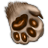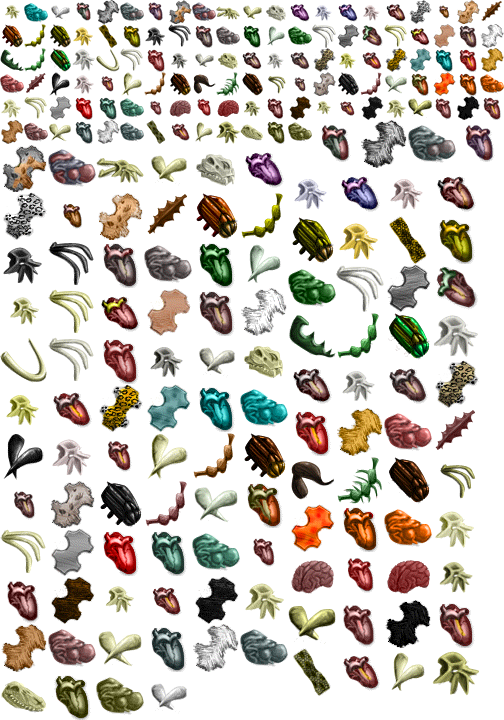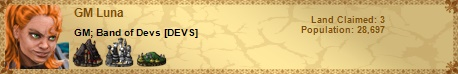Mild Release: Patch sizes, Animal parts

Category: News & Announcements
Forum Name: News & Announcements
Forum Description: Changes, patch release dates, server launch dates, downtime notifications etc.
Printed Date: 13 Jul 2020 at 04:10
Software Version: Web Wiz Forums 12.03 - http://www.webwizforums.com

Topic: Mild Release: Patch sizes, Animal parts
Posted By: GM ThunderCat
Subject: Mild Release: Patch sizes, Animal parts
Date Posted: 01 Aug 2012 at 17:43
 Animal PartsTo differentiate between animal hides and rarer animal parts on the map we have differentiated the two icons. Cotters can collect the hides, skinners the animal parts.To aid understanding of the various recipes we have added new images for the different rare animal parts:Patch Sizes:We have noticed that some mineral and herb patches were growing too large. We have now ensured that all patches are capped at the correct levels.Therefore, if you are planning a building or harvesting strategy to exploit a patch of rare resources, we would suggest that you scout out these patches afresh, as the patches that you are looking at may now not grow as large as before. This is particularly important if you are about to harvest rare herbs, as you would not want to accidentally eradicate the patch.Fixes:Trade overview now shows what all trade units are doing rather than just caravans.Cotter display of build time is now corrected.Charge can now be levelled up on the commander screen.Recalling gathers with messengers is now resolved.Bumped cotters should no longer dissipate into the void.

Replies:
Posted By: Fromfrak
Date Posted: 01 Aug 2012 at 17:55
 MMMMM, TC you are making me hungry------------- http://elgea.illyriad.co.uk/a/p/122905" rel="nofollow">Posted By: Captain Ganoes Paran
Date Posted: 01 Aug 2012 at 19:15
 thanks TC :)

Posted By: geofrey
Date Posted: 02 Aug 2012 at 18:37
 Can you list a recipe page on the site/forum? While I understand light grey skin and dark grey skin are different, I don't know what they are called and it is hard to tell them apart when not right next to each other. If no full recipe page, maybe allow a mouse over on research to list the name of the ingredient? ------------- http://elgea.illyriad.co.uk/a/p/45534" rel="nofollow">Posted By: Salararius
Date Posted: 02 Aug 2012 at 18:39geofrey wrote:Can you list a recipe page on the site/forum? While I understand light grey skin and dark grey skin are different, I don't know what they are called and it is hard to tell them apart when not right next to each other. If no full recipe page, maybe allow a mouse over on research to list the name of the ingredient?Posted By: GM Luna
Date Posted: 02 Aug 2012 at 18:55geofrey wrote:Can you list a recipe page on the site/forum? While I understand light grey skin and dark grey skin are different, I don't know what they are called and it is hard to tell them apart when not right next to each other. If no full recipe page, maybe allow a mouse over on research to list the name of the ingredient?

Players will need to discover the recipe ingredients on their own. You can get a basic idea from the mouseover, but specifics are learned via research. I'm sure once players figure all of these out they can share with eachother the recipes (and that is fine by me) but we won't be listing them anywhere at this time.

Luna

-------------Posted By: Daufer
Date Posted: 02 Aug 2012 at 19:01
 If your army kills a group of animals, will hides or animal parts spawn on the spot or is it a random spawn?

Posted By: GM Luna
Date Posted: 02 Aug 2012 at 19:03Daufer wrote:If your army kills a group of animals, will hides or animal parts spawn on the spot or is it a random spawn?

Yes if you kill a group of animals, they can essentially "drop" animal parts onto the square. You can then go harvest them.

Luna

-------------Posted By: Rill
Date Posted: 02 Aug 2012 at 19:16
 GM TC also mentioned that when animals fight each other they sometimes drop parts, which accounts for the seemingly random drops (at least partially).

Posted By: Quackers
Date Posted: 02 Aug 2012 at 19:58geofrey wrote:Can you list a recipe page on the site/forum? While I understand light grey skin and dark grey skin are different, I don't know what they are called and it is hard to tell them apart when not right next to each other. If no full recipe page, maybe allow a mouse over on research to list the name of the ingredient?

-------------
Make it your ambition to lead a quiet life, to mind your own business and to work with your hands, so that your daily life may win the respect of outsiders and so you will not be dependent on anybody.

Posted By: geofrey
Date Posted: 02 Aug 2012 at 20:41Quackers wrote:geofrey wrote:Can you list a recipe page on the site/forum? While I understand light grey skin and dark grey skin are different, I don't know what they are called and it is hard to tell them apart when not right next to each other. If no full recipe page, maybe allow a mouse over on research to list the name of the ingredient?

-------------
http://elgea.illyriad.co.uk/a/p/45534" rel="nofollow">Posted By: Salararius
Date Posted: 02 Aug 2012 at 21:27
 Harvesting caravan's do not show up as harvesting in the "Trade Overview" screen.

Posted By: Salararius
Date Posted: 02 Aug 2012 at 22:29Quackers wrote:geofrey wrote:Can you list a recipe page on the site/forum? While I understand light grey skin and dark grey skin are different, I don't know what they are called and it is hard to tell them apart when not right next to each other. If no full recipe page, maybe allow a mouse over on research to list the name of the ingredient?

How did you get that in your profile?  When I try to paste the HTML (slightly modified) in:

<div><span>Sword</span><span class="name base swords_24" data="c=3" title="Sword"></span></div>
<div><span>Bow</span><span class="name base bows_24" data="c=4" title="Bow"></span></div>
<div><span>Spear</span><span class="name base spears_24" data="c=5" title="Spear"></span></div>

it shows up in the edit window but then disappears when I press Update Profile.  I'd like to see that list with the words in front so I don't have to hover over every icon to see what it is.  I find it easier to remember the names and than the icons.

Thanks

Posted By: Quackers
Date Posted: 03 Aug 2012 at 01:06
 Updated thanks to Tallica. (copy this and past on Your Profile in game)Basic Resources[@c=183] [@c=295]  [@c=296] [@c=17] [@c=19] [@c=21] [@c=23] [@c=25]Advanced Resources[@c=1] [@c=2] [@c=3] [@c=4] [@c=5] [@c=6] [@c=7] [@c=8] [@c=9] [@c=10] [@c=11] [@c=12] [@c=420]Crafted Horses[@c=33] [@c=34] [@c=35] [@c=36] [@c=37]Crafted Swords[@c=40] [@c=41] [@c=42] [@c=43] [@c=44] [@c=46] [@c=48] [@c=49] [@c=50] [@c=51] [@c=52] [@c=53] [@c=54][@c=57] [@c=58] [@c=59] [@c=60] [@c=61] [@c=62] [@c=63] [@c=64]Crafted Bows[@c=67][@c=68][@c=69][@c=70][@c=72][@c=73][@c=74][@c=75][@c=76][@c=77][@c=78][@c=79][@c=80][@c=81][@c=82][@c=83][@c=84][@c=85][@c=86][@c=87][@c=88][@c=89]Crafted Spears[@c=91] [@c=92] [@c=93] [@c=94] [@c=95] [@c=96] [@c=97] [@c=98] [@c=99] [@c=100] [@c=101] [@c=102] [@c=103] [@c=104] [@c=105] [@c=106] [@c=107] [@c=108] [@c=109]  [@c=110] [@c=111] [@c=112] [@c=113] [@c=114] [@c=115] [@c=116] Crafted Armor[@c=120] [@c=121] [@c=122] [@c=123] [@c=124] [@c=125] [@c=126] [@c=127] [@c=128] [@c=129] [@c=130] [@c=131] [@c=132] [@c=133] [@c=134] [@c=135] [@c=136] [@c=137] [@c=138] [@c=139][@c=141] [@c=142] [@c=143] [@c=144] [@c=145] [@c=146] [@c=147] [@c=148] [@c=149] [@c=150] [@c=151] [@c=153] [@c=154] [@c=155] [@c=156] [@c=157] [@c=158] [@c=159][@c=160] [@c=161] [@c=162] [@c=163] [@c=165] [@c=166] [@c=167] [@c=168] [@c=169] [@c=170] [@c=171] [@c=172] [@c=173] [@c=174] [@c=175] [@c=176] [@c=177] [@c=178] [@c=179]Minerals[@c=417] [@c=193] [@c=194] [@c=195] [@c=196] [@c=197][@c=198] [@c=199] [@c=200][@c=201] [@c=202] [@c=203] [@c=204] [@c=205] [@c=206] [@c=207][@c=208] [@c=209] [@c=210][@c=211] [@c=212] [@c=213]Herbs[@c=416] [@c=224] [@c=225] [@c=226] [@c=227] [@c=228] [@c=229] [@c=230] [@c=231] [@c=232] [@c=233] [@c=234] [@c=235] [@c=236] [@c=237] [@c=238] [@c=239] [@c=240] [@c=241] [@c=242] [@c=243] [@c=244] [@c=245] [@c=246] [@c=247] [@c=248] [@c=249] [@c=250] [@c=251] [@c=252] [@c=253] [@c=254]Animal Parts[@c=186] [@c=298] [@c=299] [@c=300][@c=301] [@c=302] [@c=303] [@c=304] [@c=305] [@c=306] [@c=307][@c=308] [@c=309] [@c=310][@c=311] [@c=312] [@c=313] [@c=314] [@c=315] [@c=316] [@c=317][@c=318] [@c=319] [@c=320][@c=321] [@c=322] [@c=323] [@c=324] [@c=325] [@c=326] [@c=327][@c=328] [@c=329] [@c=330][@c=331] [@c=332] [@c=333] [@c=334] [@c=335] [@c=336] [@c=337][@c=338] [@c=339] [@c=340][@c=341] [@c=342] [@c=343] [@c=344] [@c=345] [@c=346] [@c=347][@c=348] [@c=349] [@c=350][@c=351] [@c=352] [@c=353] [@c=354] [@c=355] [@c=356] [@c=357][@c=358] [@c=359] [@c=360][@c=361] [@c=362] [@c=363] [@c=364] [@c=365] [@c=366] [@c=367][@c=368] [@c=369] [@c=370][@c=371] [@c=372] [@c=373] [@c=374] [@c=375] [@c=376] [@c=377][@c=378] [@c=379] [@c=380][@c=381] [@c=382] [@c=383] [@c=384] [@c=385] [@c=386] [@c=387][@c=388] [@c=389] [@c=390][@c=391] [@c=392] [@c=393] [@c=394] [@c=395] [@c=396] [@c=397][@c=398] [@c=399] [@c=400][@c=401] [@c=402] [@c=403] [@c=404] [@c=405] [@c=406] [@c=407][@c=408] [@c=409] [@c=410] [@c=411] [@c=412] [@c=413] [@c=414] [@c=415]Exotic/Elemental[@c=255] [@c=256] [@c=257] [@c=258] [@c=290] [@c=291]  [@c=292] [@c=293]DiscoverieElf: [@c=422] [@c=47] [@c=71]Human: [@c=423] [@c=65] [@c=152]Dwarf: [@c=424 ][@c=45]  [@c=164]Orc: [@c=425] [@c=55] [@c=56]Blank Code[@c=13] [@c=14] [@c=15] [@c=16] [@c=18] [@c=20] [@c=22] [@c=24] [@c=26] [@c=27] [@c=28] [@c=29] [@c=30] [@c=31] [@c=32] [@c=38] [@c=39] [@c=66] [@c=117] [@c=118] [@c=119] [@c=140] [@c=180] [@c=181] [@c=182] [@c=184] [@c=185] [@c=187] [@c=188] [@c=189] [@c=190] [@c=191] [@c=192] [@c=214] [@c=215] [@c=216] [@c=217] [@c=218] [@c=219] [@c=220] [@c=221] [@c=222] [@c=223] [@c=259] [@c=260][@c=261] [@c=262] [@c=263] [@c=264] [@c=265] [@c=266] [@c=267] [@c=268] [@c=269] [@c=270] [@c=271] [@c=272] [@c=273] [@c=274] [@c=275] [@c=276] [@c=277] [@c=278] [@c=279] [@c=280] [@c=281] [@c=282] [@c=283] [@c=284] [@c=285] [@c=286] [@c=287] [@c=288] [@c=289] [@c=294] [@c=297] [@c=418] [@c=419] [@c=421] -------------Make it your ambition to lead a quiet life, to mind your own business and to work with your hands, so that your daily life may win the respect of outsiders and so you will not be dependent on anybody.

Posted By: Makanalani
Date Posted: 03 Aug 2012 at 01:38
 Where do you find your skinners because my skinner guild and marketplace do not have them?-------------"Life is a beautiful struggle"-New IGN: Mak (Dark Blight)

Posted By: Quackers
Date Posted: 03 Aug 2012 at 02:03Makanalani wrote: Where do you find your skinners because my skinner guild and marketplace do not have them?

Skinners show up in marketplace after you research butchery

-------------
Make it your ambition to lead a quiet life, to mind your own business and to work with your hands, so that your daily life may win the respect of outsiders and so you will not be dependent on anybody.

Posted By: Salararius
Date Posted: 03 Aug 2012 at 02:08
 Thanks so much, here is what I was looking for but what you posted had what I didn't know in it. http://elgea.illyriad.co.uk/#/Player/Profile/66699" rel="nofollow - http://elgea.illyriad.co.uk/#/Player/Profile/66699 Thanks!

Posted By: Quackers
Date Posted: 03 Aug 2012 at 02:16Salararius wrote:Thanks so much, here is what I was looking for but what you posted had what I didn't know in it. http://elgea.illyriad.co.uk/#/Player/EditProfile/66699" rel="nofollow - http://elgea.illyriad.co.uk/#/Player/EditProfile/66699 Thanks!

Yea I wanted to give you the code I typed but then I noticed all the blank code that did nothing. Didn't want you to have to shift through and figure all that stuff out as well. :P

-------------
Make it your ambition to lead a quiet life, to mind your own business and to work with your hands, so that your daily life may win the respect of outsiders and so you will not be dependent on anybody.

Posted By: lokifeyson
Date Posted: 03 Aug 2012 at 06:50
 dude yes, just made me like the update more :)i was saying we needed exactly this :)-------------Posted By: tallica
Date Posted: 18 Aug 2012 at 21:13
 Quackers missed a couple of crafted weapons (and maybe armors?) which I've included in this list: (you can copy this to wherever you'd like)Basic Resources[@c=183][@c=17][@c=19][@c=21][@c=23][@c=25][@c=295][@c=296]Advanced Resources[@c=1][@c=2][@c=12][@c=7][@c=5][@c=3][@c=4][@c=6][@c=8][@c=9][@c=10][@c=11][@c=420]Minerals[@c=417][@c=193][@c=194][@c=195][@c=196][@c=197][@c=198][@c=199][@c=200][@c=201][@c=202][@c=203][@c=204][@c=205][@c=206][@c=207][@c=208][@c=209][@c=210][@c=211][@c=212]Herbs[@c=416][@c=253][@c=224][@c=225][@c=226][@c=227][@c=228][@c=229][@c=230][@c=231][@c=232][@c=233][@c=234][@c=235][@c=236][@c=237][@c=238][@c=239][@c=240][@c=241][@c=242][@c=243][@c=244][@c=245][@c=246][@c=247][@c=248][@c=249][@c=250][@c=251][@c=252]Animal Parts[@c=186][@c=298][@c=299][@c=300][@c=301][@c=302][@c=303][@c=304][@c=305][@c=306][@c=307][@c=308][@c=309][@c=310][@c=311][@c=312][@c=313][@c=314][@c=315][@c=316][@c=317][@c=318][@c=319][@c=320][@c=321][@c=322][@c=323][@c=324][@c=325][@c=326][@c=327][@c=328][@c=329][@c=330][@c=331][@c=332][@c=333][@c=334][@c=335][@c=336][@c=337][@c=338][@c=339][@c=340][@c=341][@c=342][@c=343][@c=344][@c=345][@c=346][@c=347][@c=348][@c=349][@c=350][@c=351][@c=352][@c=353][@c=354][@c=355][@c=356][@c=357][@c=358][@c=359][@c=360][@c=361][@c=362][@c=363][@c=364][@c=365][@c=366][@c=367][@c=368][@c=369][@c=370][@c=371][@c=372][@c=373][@c=374][@c=375][@c=376][@c=377][@c=378][@c=379][@c=380][@c=381][@c=382][@c=383][@c=384][@c=385][@c=386][@c=387][@c=388][@c=389][@c=390][@c=391][@c=392][@c=393][@c=394][@c=395][@c=396][@c=397][@c=398][@c=399][@c=400][@c=401][@c=402][@c=403][@c=404][@c=405][@c=406][@c=407][@c=408][@c=409][@c=410][@c=411][@c=412][@c=413][@c=414][@c=415][@c=290][@c=291][@c=292][@c=293][@c=255][@c=256][@c=257][@c=258]Swords[@c=40][@c=41][@c=42][@c=43][@c=44][@c=45][@c=46][@c=47][@c=48][@c=50][@c=51][@c=52][@c=53][@c=54][@c=55][@c=56][@c=57][@c=58][@c=59][@c=60][@c=61][@c=62][@c=63][@c=64][@c=65][@c=49]Bows[@c=67][@c=68][@c=69][@c=70][@c=71][@c=72][@c=73][@c=74][@c=75][@c=76][@c=77][@c=78][@c=79][@c=80][@c=81][@c=82][@c=83][@c=84][@c=85][@c=86][@c=87][@c=88][@c=89]Spears[@c=91][@c=92][@c=93][@c=94][@c=95][@c=96][@c=97][@c=98][@c=99][@c=100][@c=101][@c=102][@c=103][@c=104][@c=105][@c=106][@c=107][@c=108][@c=109][@c=110][@c=111][@c=112][@c=113][@c=114][@c=115][@c=116]Horses[@c=33][@c=34][@c=35][@c=36][@c=37][@c=422][@c=423][@c=424][@c=425]Leather Armors[@c=120][@c=121][@c=122][@c=123][@c=124][@c=125][@c=126][@c=127][@c=128][@c=129][@c=130][@c=131][@c=132][@c=133][@c=134][@c=135][@c=136][@c=137][@c=138][@c=139]Chain Armors[@c=141][@c=142][@c=143][@c=144][@c=145][@c=146][@c=147][@c=148][@c=149][@c=150][@c=151][@c=152][@c=153][@c=154][@c=155][@c=156][@c=157][@c=158][@c=159]Plate Armors[@c=161][@c=162][@c=163][@c=164][@c=165][@c=166][@c=167][@c=168][@c=169][@c=170][@c=171][@c=172][@c=173][@c=174][@c=175][@c=176][@c=177][@c=178][@c=179]

Posted By: Salararius
Date Posted: 18 Aug 2012 at 22:18
 Which ones? :) Ah, found them.Thank You

Posted By: tallica
Date Posted: 18 Aug 2012 at 22:29
 mine has a couple of weapons that were missing from Quackers, so the 2nd one! Edit: or are you asking for which ones were missed? That question I don't know anymore, spend so long going through them all!

Posted By: dunnoob
Date Posted: 19 Aug 2012 at 03:07to Mogul, Quackers, Salarius, and Tallica; I've copied the list in an IGM.  Is it only me, or does everybody send lots of IGMs to themselves for archiving?

Posted By: tallica
Date Posted: 19 Aug 2012 at 17:03dunnoob wrote:to Mogul, Quackers, Salarius, and Tallica; I've copied the list in an IGM.  Is it only me, or does everybody send lots of IGMs to themselves for archiving?

That's what I did with my set, well, just the crafting items so that I have a quick reference at hand.

Posted By: Wondering
Date Posted: 19 Aug 2012 at 20:36
 The game icons are in sprite format, which while great for game speed, makes it difficult for 3rd party tools and fansites.Can individual icons be created for player use, perhaps added to the updated fansite package?

Posted By: Elf Dragon
Date Posted: 19 Aug 2012 at 21:25
 Thanks for that :)

Posted By: abstractdream
Date Posted: 19 Aug 2012 at 21:40
 I want to thank you ALL for your dedication and hard work. The list is quite useful and I go back to it almost every day.I bookmark the IGM with the codes for quick reference. Thanks again. -------------Bonfyr Verboo

 Print Page | Close Window Forum Software by Web Wiz Forums® version 12.03 - http://www.webwizforums.comCopyright ©2001-2019 Web Wiz Ltd. - https://www.webwiz.net